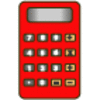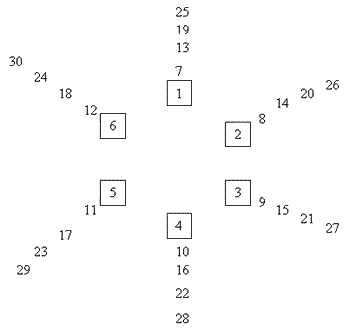#### You may also like### Biscuit Decorations

Andrew decorated 20 biscuits to take to a party. He lined them up and put icing on every second biscuit and different decorations on other biscuits. How many biscuits weren't decorated?### Constant Counting

You can make a calculator count for you by any number you choose. You can count by ones to reach 24. You can count by twos to reach 24. What else can you count by to reach 24?### Number Detective

Follow the clues to find the mystery number.

# Six in a Circle

##### Age 5 to 7 Challenge Level:

We received two particularly good solutions to this problem. The first is from Helen and Daniela who go to Aldermaston C of E Primary School. They drew this table to help answer the questions:

 1 2 3 4 5 6 7 8 9 10 11 12 13 14 15 16 17 18 19 20 21 22 23 24 25 26 27 28 29 30

They therefore concluded that:
There are $4$ children behind each chair.
Children $9, 15, 21, 27$ were standing behind chair $3$.
Here's the table they drew for only four chairs:

 1 2 3 4 5 6 7 8 9 10 11 12 13 14 15 16 17 18 19 20 21 22 23 24 25 26 27 28 29 30

They comment that:
Seven children are behind $1$ and $2$. Six children are behind $3$ and $4$.
There can't be the same number behind each chair because $30$ doesn't go into $4$ but $30$ goes into $6$. (I think perhaps you mean that $4$ doesn't go into $30$, but $6$ does.)

The second was from Joe and Richard at St. Nicolas CE Junior School, Newbury who also drew a diagram to help them solve the problem:Joe and Richard say:
We started by drawing children round the circle like in our diagram above. We answered the questions next.
1) Four people were standing behind each chair.
2) Behind number $3$, there were $9, 15, 21, 27$.

We made another drawing but with $4$ chairs and put the children round the circle. In the end, we counted the children up. $1$ and $2$ had $7$ children behind each and $3$ and $4$ had $6$ children. Then we noticed that however many chairs there were, the numbers went up by the highest numbered chair. We tested this solution by using $2$ to $6$ chairs and it worked.

We also noticed that on the last chair, the numbers go up in its table. We tested this on numbers of chairs from $2$ to $6$ and it worked with every single one.

We noticed that because $6$ is a factor of $30$ and $4$ isn't, $4$ didn't have the same number of children around each chair and $6$ did. Then we noticed the only numbers of chairs that would have an equal number of children behind each chair were factors of $30$, which are $1, 2, 3 ,5, 6, 10, 15$ and $30$.

Helen and Pauline from Moorfield Junior School also drew a chart. I think this was a useful strategy. Well done to everyone who answered this correctly and gave good explanations.